# Area of a Triangle Worksheet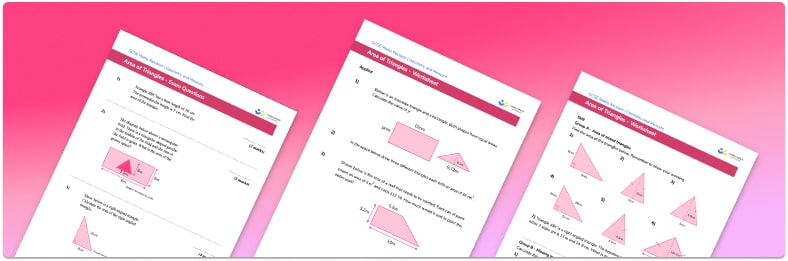• Section 1 of the area of a triangle worksheet contains 7 skills-based area of a triangle questions, in 3 groups to support differentiation
• Section 2 contains 3 applied area of a triangle questions with a mix of worded problems and deeper problem solving questions
• Section 3 contains 2 foundation and higher level GCSE exam style questions
• Answers and a mark scheme for all questions are provided
• Questions follow variation theory with plenty of opportunities for students to work independently at their own level
• All questions created by fully qualified expert secondary maths teachers
• Suitable for GCSE maths revision for AQA, OCR and Edexcel exam boards
• Other printable worksheets, including area of a parallelogram, area of compound shapes and area of trapezoid, are available

• This field is for validation purposes and should be left unchanged.

You can unsubscribe at any time (each email we send will contain an easy way to unsubscribe). To find out more about how we use your data, see our privacy policy.

### Area of a triangle at a glance

The formula for the area of a triangle is derived from the formula for the area of a rectangle. To work out the area of a triangle, we use the formula ½ base perpendicular height. This formula works for all types of triangles, including equilateral triangles, isosceles triangles and right triangles. Area is the amount of space covered by a 2D shape and is measured in square units – metric units of area include square millimetres, square centimetres and square metres.

Sometimes students are given the total area of the triangle, and are asked to find the height of the triangle or the length of the base of the triangle. To do this, we need to divide the area by the given side length. This can require students to work with decimals so it is important these skills are secure. They may also need to convert between different metric units – for example, if one side is given in centimetres and the other in millimetres.

Compound shapes are made from two or more simple shapes, such as a quadrilateral with a triangle on top. To find the area of compound shapes, we break into the component parts and find the area of each shape.

In addition to using integers for the sides of the triangle, decimals and fractions are also common when finding an area. Students may also be asked to apply multiplication of fractions to the area of a triangle. When tackling word problems on the area of a triangle, students may have to apply these skills to problems such as finding the area of a room or garden space, and then apply additional skills such as calculating a cost to cover or pave that space.

Looking forward, students can then progress to additional area worksheets and other geometry worksheets, for example an angles in polygons worksheet or area and circumference of a circle worksheet.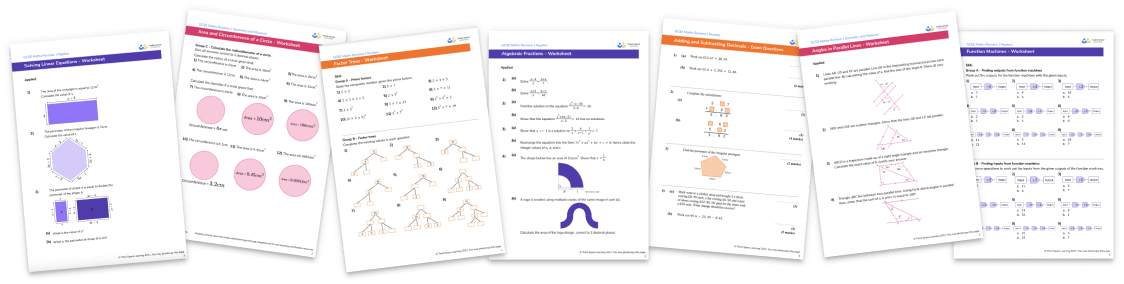For more teaching and learning support on Geometry our GCSE maths lessons provide step by step support for all GCSE maths concepts.

## Related worksheets

Area of a Circle Worksheet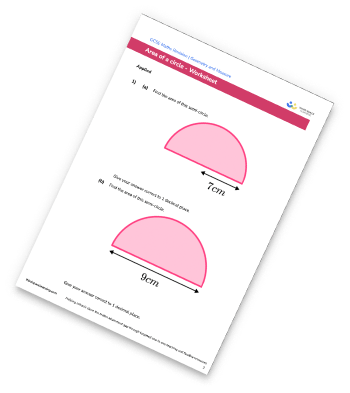Area of Compound Shapes Worksheet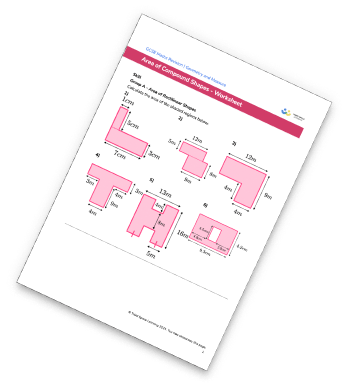Area Of A Trapezium Worksheet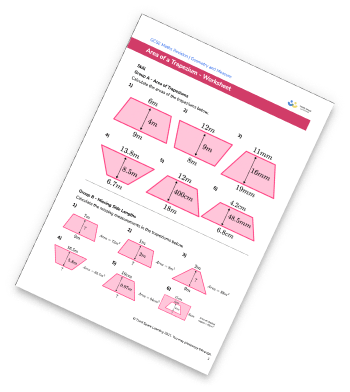Area Of A Rhombus Worksheet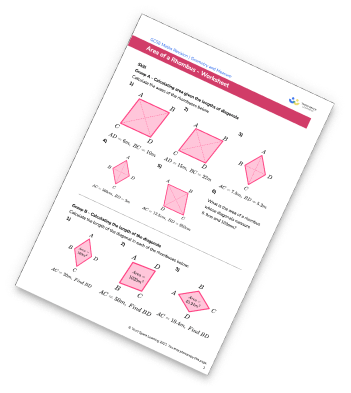## Do you have KS4 students who need more focused attention to succeed at GCSE?There will be students in your class who require individual attention to help them succeed in their maths GCSEs. In a class of 30, it’s not always easy to provide.

Help your students feel confident with exam-style questions and the strategies they’ll need to answer them correctly with our dedicated GCSE maths revision programme.

Lessons are selected to provide support where each student needs it most, and specially-trained GCSE maths tutors adapt the pitch and pace of each lesson. This ensures a personalised revision programme that raises grades and boosts confidence.

Find out more